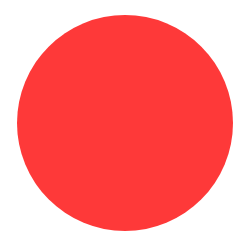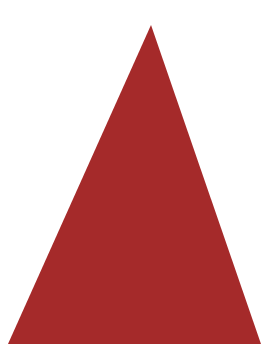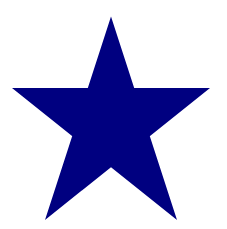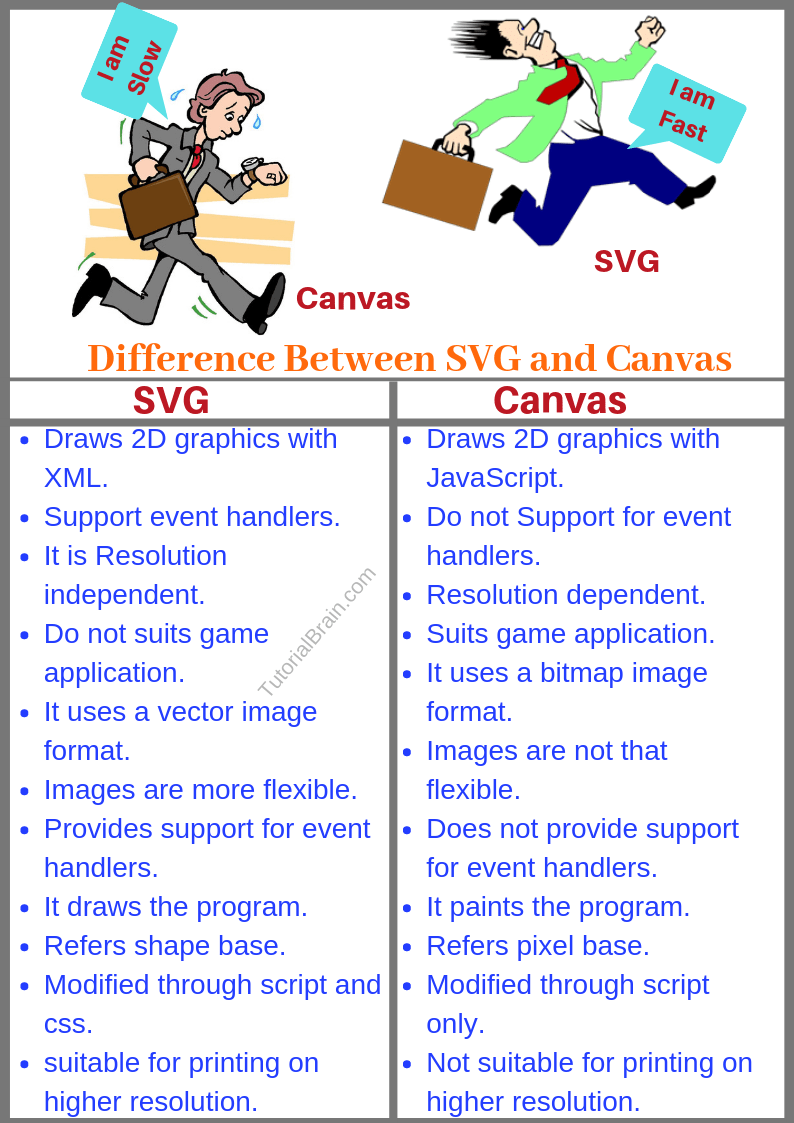# HTML5 SVG

## Example of SVGByTonchino - Own work, CC BY-SA 3.0, Link
##### What is SVG in HTML5?

HTML5 SVG is an alternative to Canvas. In simple terms, SVG helps in creating 2 Dimensional vector graphics for the website. The full form of SVG is Scalable Vector Graphics and it depicts the image or its related object in XML format which is like a special text format.

Vector Graphics are made using line, points, and arc by using some mathematical formula. It is also a W3C recommendation.

Along with SVG, the other file formats which use Vector Graphics are PDF and EPS.

On the other hand, Images like PNG, GIF and JPEG uses Raster Graphics. Raster Graphic is also called as Bitmap Image, and they use pixels(set of dots), unlike Vector Graphics.

## Vector Graphics VS Raster Graphics

 Vector Graphics Raster Graphics Consists of XML format using line, points & arc. Consists of Pixels format like dots. Smaller in size. Bigger in size than vector graphics. If you Zoom in the image, the quality of the image is not lost after scaling. The quality of the image is lost after zooming. Examples of Vector graphics are SVG, PDF and EPS. Examples of Raster graphics are PNG, APNG, JPEG, MPEG4, and GIF. Famous tools based on Vector Graphics are Adobe Illustrator & CorelDRAW. Famous tools based on Raster Graphics is Adobe Photoshop. When to use – Use this for Logo Design, Line art, Graphs, Flyers, Business Card, Brochure etc When to use – Use this for photos, collage, Background image etc. Good for print Not so good for Print compared to Vector

In the below Image, you will notice that if you zoom in a Raster Image like PNG, JPEG or GIF, then the image becomes blurry and the quality is reduced while Vector Image like SVG does not have an impact on its quality even on Zoom in.

### Vector(like SVG) VS Raster (like PNG, GIF and JPEG)By The original uploader was Darth Stabro at English Wikipedia. - Transferred from en.wikipedia to Commons by Pbroks13 using CommonsHelper., CC BY-SA 3.0, Link

## Advantages of HTML5 SVG - Why to use

• If you scale the SVG image, it will not lose its quality at any resolution
• Normally, the image size is smaller
• You can modify the image using JavaScript
• You can also animate the SVG image using animation

## How to create SVG on a web page

To embed SVG inside a web page, you can use the <svg> tag.

Using this tag, you can hold the SVG graphics so it is the container for SVG.

## SVG Text

To draw HTML5 SVG containing Texts, you should use the <text> element inside your SVG.

Set these attributes –

• x – The x-coordinate of the starting point of the text
• y – The y-coordinate of the starting point of the text
• Setting the width and height of the SVG is optional but often a good idea

Syntax:
<svg>
<text x=”x-pos” y=”y-pos”> Text…
</text>
</svg>

## Example

```<svg height="50" width="300" >
<text x="20" y="35" fill="green">Example for SVG Text</text>
</svg>
```

## HTML5 SVG Rectangle

To draw a Rectangle inside SVG, you should use the <rect> element inside your SVG.

Set these attributes –

• x – The x-coordinate of the top left corner
• y – The y-coordinate of the top left corner
• width – The width of the rectangle
• height – The height of the rectangle

In the below example, the <fill> is optional. This sets the color of the rectangle as darkred.Syntax:
<svg>
<rect x=”x-top-left” y=”y-top-left” width=”w-val” height=”h-val” />
</svg>

## Example

```<svg height="300" width="300">
<rect x="20" y="20" width="200" height="100" fill="darkred" />
</svg>
```

## SVG Rectangle With Text

Now, we are going to combine the <rect> element with the <text> element to create text inside the rectangle of SVG.## Example

```<svg height="300" width="300">
<rect x="20" y="20" width="300" height="100" />
<text x="70" y="70" fill="gold" font-weight="bold">SVG Rectangle With Text</text>
</svg>
```

## SVG Rounded Rectangle

Just code the <rx>, <ry> or both the elements to create rounded corners for X point and y point respectively.## Example

```.flexbox-container17aa {
<svg height="300" width="300">
<rect x="20" y="20" width="200" height="100" rx="20" fill="purple" />
</svg>
```

## SVG Circle

To draw a circle inside HTML SVG, you should use the <circle> element inside your SVG.

Inside this element, you need to set these attributes –

• cx – The x-coordinate of the center of the circle
• cy – The y-coordinate of the center of the circle
• r – The radius of the circle

In the below example, the radius of the circle is 30% relative to the SVG so if you change the width and height values of SVG, the area of the circle will also change.

To fix the radius irrespective of the SVG width and height values, you can use a fixed value of radius like r:”120″.Syntax:
<svg>
</svg>

## Example

```<h2>Example for SVG Circle </h2>
<svg height="400" width="400">
<circle cx="180" cy="180" r="30%" fill="#fe3939" />
</svg>
```

## HTML5 SVG Circle With Text

Let us combine the <circle> and <text> element to create text inside the circle of SVG.## Example

```<h2>Example of SVG Circle with Text </h2>
<svg height="400" width="400">
<circle cx="120" cy="120" r="30%" fill="#117da9" />
<text x="50" y="120" fill="white">SVG circle with text</text>
</svg>
```

## SVG Half Circle

Can you create a half circle inside the SVG?

Just set the width and height of the SVG and keep on changing the values of cx, cy and r values in such a way that the value of cx, cy and r should not be enough to create a full circle.

## Example

```<svg height="400" width="100">
<circle cx="120" cy="120" r="30%" fill="navy" />
</svg>
```

## SVG Stroke

The stroke property adds a stoke color(outline color). Optionally, you can use the stroke-width property to set the width of the stroke.

Syntax:
<svg stroke=”stroke-color” stroke-width=”s-width”>
<SVG elements…>
</svg>

## Example

```<h2>Example of SVG Stroke</h2>
<svg width="200" height="200" stroke-width="10" stroke="orange">
<circle cx="80" cy="80" r="60"/>
</svg>
```

## SVG Line

The <line> element creates line inside your SVG.

Set these attributes –

• x1 – The x-coordinate of the starting point of a line
• y1 – The y-coordinate of the starting point of a line
• x2 – The x-coordinate of the ending point of a line
• y2 – The y-coordinate of the ending point of a line
• stroke – To create a stroke

Syntax:
<svg>
<line x1=”x-start-pos” y1=”y-start-pos”
x2=”x-end-pos” y2=”y-end-pos”
stroke=”crimson”/>
</svg>

## Example

```<svg height="50" width="300">
<line x1="250" y1="30" x2="15" y2="30" stroke-width="10" stroke="crimson"/>
</svg>
<h2>Example of SVG dasharray line </h2>
<svg height="150" width="300">
<line x1="250" y1="30" x2="15" y2="30" stroke-width="10" stroke="purple"
stroke-dasharray="5,5"/>
</svg>
```

## SVG Paths

HTML5 SVG Path is a very detailed topic in itself.

The commands below are in Capital letter as well as small letters. The Capital letters will be for absolute position while the lower letters will be for relative positionHere is list of SVG Paths Commands and Description

M/m
moveto – to set a new initial point and a moving current point. You must code the moveto at the start of the first data segment. There can be multiple moveto to start another subpath. Parameters – (x y)+

L/l
lineto – Draw a line using (x,y) coordinate. Parameters – (x y)+

H/h
Horizontal lineto – Draw a horizontal line using x coordinate. Parameters – x+

V/v
Vertical lineto – Draw a vertical line using y coordinate. Parameters – y+

C/c
curveto – Draw cubic Bézier curve. Parameters – (x1 y1 x2 y2 x y)+

S/s
shorthand/smooth curveto – Draw smooth cubic Bézier curve. Parameters – (x2 y2 x y)+

Q/q
quadratic Bézier curveto. Parameters – (x1 y1 x y)+

T/t
shorthand/smooth quadratic Bézier curveto. Parameters – (x y)+

A/a
elliptical arc – To draw an elliptical curve using x-axis, y-axis, rx, ry,
rotation, large-arc-flag, and sweep-flag. Parameters – (rx ry x-axis-rotation large-arc-flag sweep-flag x y)+

Z/z
closepath – To close the SVG path. Parameters – None

## Example of SVG Path

```<svg width="300" height="220" stroke-width="10" stroke="orange">
<path d="M350 250 L360 120 H250 -30 V0 10"/>
</svg>
<hr>
<svg width="300" height="220" stroke-width="10" stroke="orange">
<path d="M 50 80 Q 195 10 80 180"/>
</svg>
```

Using the above commands, let us create another SVG path or arc.

## Example

```<h2>Example for SVG Arc </h2>
<svg height="200" width="300">
<path d="M150 -50 A30 50 -50 0 10 100 0" stroke="grey" stroke-width="10" fill="none"/>
</svg>
<hr>
<svg height="400" width="400">
<path d="M250 150 A30 50 50 10 80 90 0" stroke="crimson" stroke-width="10" fill="none"/>
</svg>
```

## SVG Rotate

To rotate the element inside the SVG, we can use the transform=”rotate(x, y, angle)”.

Syntax:
<svg>
<element… transform=”rotate(x, y, angle)”/>
</svg>
;

## Example

```<svg width="300" height="300">
<rect x="50" y="50" width="100" height="150" transform="rotate(60, 100, 180)"/>
</svg>
```

## SVG Fallback

All the modern browsers support SVG but some old browsers might not support it so you should code a fallback typically in form of text to display the text for those browsers who do not support SVG.

In the example below, the text “Your browser does not support SVG elements” will be displayed in the browser in case your browser does not support SVG.

## Example

```<svg height="350" width="400">
Your browser does not support Svg elements
<circle cx="50" cy="50" fill="coral" r="50" />
</svg>
```

## SVG Image

The HTML5 SVG <image> element can embed the Raster graphical image inside the SVG image. A Raster graphic is also called a Bitmap image like PNG, APNG, JPEG, GIF & MPEG4.

Syntax:
<svg>
</svg>

## Example

```<svg width="400" height="300">
<image x="30" y="40" xlink:href="https://i.ibb.co/6WKgPPZ/bird-one.png" width="400" height="250"/>
</svg>
```

## HTML5 SVG Triangle

The SVG <polygon> element helps to create polygon objects like triangle, star, pentagon, hexagon, octagon, etc.

Suppose, you wish to create an SVG triangle, then you should set the x & y coordinates of 1st, 2nd and 3rd angles of the triangle.Syntax:
<svg>
<polygon points=”x1,y1  x2,y2  x3,y3″/>
</svg>

## Example

```<svg height="400" width="400">
<polygon points="250,60 120,350 350,350" fill="brown" />
</svg>
```

## SVG Star

Let us create a star using the SVG <polygon> element. In this case, you have to set the x & y coordinate of the 1st, 2nd,  3rd, 4th and 5th angle.Syntax:
<svg>
<polygon points=”x1,y1  x2,y2  x3,y3
x4,y4  x5,y5 “/>
</svg>

## Example

```<svg height="300" width="300">
<polygon points="100,15 40,200 190,80, 10,80 160,200" fill="navy" />
</svg>
```

Now, we are going to create multiple SVG Polygon objects like a pentagon, Hexagon, and Octagon.

To create Pentagon, set the x,y coordinates of 5 angles.

For Hexagon, set the x,y coordinates of 6 angles.

And for Octagon, we need set x,y coordinates of 8 angles.## Example

```<h2>Example of SVG POLYGONS </h2>
<h3>SVG Pentagon</h3>
<svg height="120" width="300">
<polygon points="50 3, 100 38, 82 100, 20 100, 3 38" stroke="red" stroke-width="5" />
</svg>
```

## SVG Polyline

The meaning of Poly is multiple so Polyline means multiple connected lines.

It is easy to create SVG Polyline using the <polyline> element. You must set the x & y coordinate of each connected multiple points of the line.

Syntax:
<svg>
<polyline points=”x1,y1  x2,y2  …
xn,yn“/>
</svg>

## Example

```<svg height="120" width="300">
<polyline points="150 50, 250 18, 182 100, 10 50" fill="none" stroke="green" stroke-width="5" />
</svg>
```

## SVG Ellipse​

The SVG <ellipse> element create ellipse.

To create the SVG, you must set the center of the ellipse and the radius along x and y axis.

Set these attributes –

• cx – X-coordinate of the center of the ellipse
• cy – Y-coordinate of the center of the ellipse
• rx – Radius of the ellipse along X axis
• ry – Radius of the ellipse along Y axisSyntax:
<svg>
<ellipse cx=”x-center” cy=”y-center”
</svg>

## Example

```<h2>Example of SVG ELLIPSE </h2>
<svg height="200" width="300">
<ellipse cx="150" cy="100" rx="50" ry="80" fill="dodgerblue" stroke="black" stroke-width="5"/>
</svg>
```

The SVG element can help to create a smooth texture of colors in a linear way which is called a linear SVG gradient. This must contain an id attribute to refer it later.

We can create a vertical gradient as well as a horizontal gradient. Another way is to create a SVG gradient in the same direction as to how SVG is defined.

The must always be nested inside the element. The element helps to embed the properties and definition of an SVG object that can be reused inside an SVG object.

There are 2 types which decide the stopping offset position to create linear SVG gradients. Each contains a to apply a particular color.

Optionally, you can set for each to set the opacity between the stop points.

It is better to set these attributes for the element –

• x1 – X-coordinate of the starting point of the SVG to define the SVG linear gradient
• y1 – Y-coordinate of the starting point of the SVG to define the linear gradient
• x2 – X-coordinate of the end point of the SVG to define the linear gradient
• y2 – Y-coordinate of the end point of the SVG to define the linear SVG gradient

Similarly, the radial gradient creates textures of colors in a circular way. The SVG is used for this purpose. This element should also contain an id attribute to refer it later. In this case –

• x1 – X-coordinate of the center of the SVG radial gradient
• x2 – X-coordinate of the focal point of the radial gradient

## Example

```<svg height="250" width="300" >
<defs>
<stop offset="25%"  stop-color="cyan" stop-opacity="0.5" />
<stop offset="75%" stop-color="dodgerblue" />
</defs>
<rect x="50" y="20" width="200" height="200" rx="30" fill="url(#gradval)"/>
</svg>
```

## SVG FiltersThe SVG <filter> element is used to create effects like blur effect, highlight effect, drop shadow effect, morphed image, color matrix etc.

The <filter> element must be present inside the <defs> element which in turn is wrapped inside the <svg> element.

Inside the <filter> element, you can use any of these filters –

• feMorphology – Create a morphed image
• feColorMatrix – The color matrix helps to create a color matrix on the SVG image
• feGaussianBlur – The Gaussian Blur can be used to blur the SVG image

The stdDeviation controls the density of the filter. For example – If you want to apply a blur effect and you have a higher number for stdDeviation, then the SVG will have more blur effect.

The result attribute is optional. It is the output of the filter and can act as an input to any other filter.

## Example

```<h2>Example of SVG Filters </h2>
<svg height="220" width="400" >
<defs>
</filter>
</defs>
</svg>
```

Let us blur an SVG object using the feGaussianBlur.

## Example

```<svg height="300" width="600" >
<defs>
<filter id="rectblur">
<feGaussianBlur in="SourceGraphic" stdDeviation="5"></feGaussianBlur>
</filter>
</defs>
<rect x="50" y="20" width="150" height="150" rx="30" fill="hotpink"/>
<rect x="250" y="20" width="150" height="150" rx="30" fill="hotpink" filter="url(#rectblur)"/>
</svg>
```

## SVG Clip Path

The SVG <clipPath> is used to clip an SVG object based on the path which it selects. The image inside the path is visible while the clipped part is not visible.

This element must contain an id attribute to refer it for clipping.## Example

```<svg class="svg-graphic" width="400" height="300">
<g>
<clipPath id="clipimg">
<polygon points="150 0, 250 150, 50 200, 0 100" />
</clipPath>
</g>
<image clip-path="url(#clipimg)" height="300" width="300" xlink:href="https://i.ibb.co/YkTLTng/dog.png" />
</svg>
```

The SVG <mask> element is similar to the clip path that creates a mask on the SVG object. This always contains an id attribute

Now, using the mask id, another SVG object/image can use this mask.

```<svg>
<rect x="0" y="0" width="200" height="200" fill="white" />
<polygon points="150,130 20,30 100,30" fill="brown" />
<polygon points="15 13, 120 38, 60 100, 20 10, 13 58" fill="crimson" />
</svg>
```

## SVG Animation

Animate the SVG object, using the following elements –

• <animate> – To animate a particular property of SVG object
• <animateTransform> – To animate the transform property of SVG element
• <animateMotion> – To animate along the motion i.e. follow along the path of the motion
In the example below, the attributeName is x so, this will change the x value of the rectangle from 10 to 100 in a duration of 3 seconds and will repeat it for an infinite number of times. To limit it to 10 times, you can set repeatCount=”10″.

Try to change the attributeName as y or width or height etc and see the difference.

## Example

```<svg width="300" height="100">
<rect id="svganimation" width="250" height="70" x="20" y="20" stroke-width="5" stroke="red" fill="navy" />
<animate xlink:href="#svganimation" attributeName="x" from="20" to="250" dur="3s" repeatCount="indefinite" />
</svg>
```

## SVG Viewbox

The SVG <viewbox> can be used to redefine the coordinates inside an SVG.

Suppose, the initial width of the SVG object is 340px and height is 240px.

Now, let’s draw a rectangle inside the SVG whose width is 40 and height is 30px and x,y is 5,3.

Now, if you set –
viewbox: 0 0 68 48 on the SVG, that means the x & y coordinate of the viewbox are 0 each. The width of viewbox is 10px while height of the viewbox is 20px.

This means 1 unit of coordinate inside SVG will occupy 340/68 =5px width and 240/48 = 5px height.

So, indirectly x coordinate of the rectangle will be at 40*5 = 200px while y coordinate of the rectangle will be at 30*5=150px.

## Example

```<svg viewBox="0 0 68 48" height="240" width="340">
<rect x="5" y="3" width="40" height="30" fill="darkred" />
</svg>
```

## SVG Fill Color With CSS

You can style the SVG object using CSS(Cascading Style Sheets).

You can style the properties of SVG like <fill>, <stroke> etc using CSS.

If you do not know about CSS, then click on this link which will lead you to out CSS Tutorial Page.

## Example

```<svg width="300" height="300">
<circle cx="140" cy="140" r="30%"/>
</svg>
```

## Difference between HTML Canvas and SVG